Courses

# Test: Design of Bearings Level - 2

## 30 Questions MCQ Test Engineering Mechanics | Test: Design of Bearings Level - 2

Description
This mock test of Test: Design of Bearings Level - 2 for Mechanical Engineering helps you for every Mechanical Engineering entrance exam. This contains 30 Multiple Choice Questions for Mechanical Engineering Test: Design of Bearings Level - 2 (mcq) to study with solutions a complete question bank. The solved questions answers in this Test: Design of Bearings Level - 2 quiz give you a good mix of easy questions and tough questions. Mechanical Engineering students definitely take this Test: Design of Bearings Level - 2 exercise for a better result in the exam. You can find other Test: Design of Bearings Level - 2 extra questions, long questions & short questions for Mechanical Engineering on EduRev as well by searching above.
QUESTION: 1

### The most suitable bearing for carrying very heavy loads with slow speed is

Solution:

In hydrostatic bearing, there is no metal to metal contact, even at low speed.

QUESTION: 2

Solution:
QUESTION: 3

### A ball bearing is characterized by basic static capacity = 11000 N & dynamic capacity = 18000 N. This bearing is subjected to equivalent static load =5500 N . The bearing loading ratio and life in million revolutions respectively are

Solution: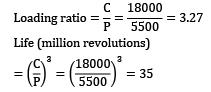QUESTION: 4

Which one of the following is correct?

A hydrodynamic slider bearing develops load bearing capacity mainly because of

Solution:

A hydrodynamic slider bearing develops load bearing capacity mainly because of wedge shaped oil film.

QUESTION: 5

A full journal bearing having clearance to radius ratio of 1/100, using a lubricant with μ = 22 X 10-3 as supports the shaft journal running at N = 2400 r.p.m. If the bearing pressure is 1.4 MPa, the Sommerfeld’s number is

Solution: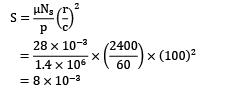= 8 x 10-3

QUESTION: 6

What is the main advantage of hydrodynamic bearing over roller bearing?

Solution:

Hydrodynamic bearing have more load carrying capacity as compared to roller bearing.

QUESTION: 7

Match List-I (Type of Anti-friction bearing) with List-II (Specific Use) and select the correct answer using the code given below the Lists: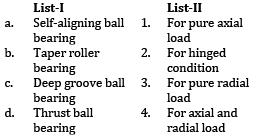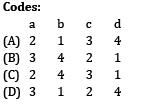Solution:
QUESTION: 8

A roller bearing has a dynamic load capacity of 30 kN and radial load acting on bearing is 5 kN. The desired life for 90% of the bearing is 10000 hr. What is the speed (in rpm) at which bearing is working?

Solution:

Life of bearing in million revolutions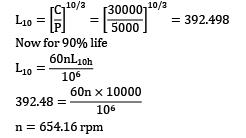Now for 90% lifen = 654.16 rpm

QUESTION: 9

A full journal bearing have clearance to radius ratio of 1/200. The lubricant with μ = 40 X 10-3 Pa-s supports the shaft journal running at 3000 rpm. If the bearing pressure is 1.5 MPa, the Sommerfeld’s number is

Solution: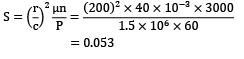= 0.053

QUESTION: 10

To restore stable operating condition in a hydrodynamic journal bearing, when it encounters higher magnitude loads,

Solution:

In hydrodynamic bearing if the load increases, then bearing characteristic number (μN/p ) decreases and the bearing will move towards unstable region (thin lubrication). So to restore stable condition the bearing characteristic number is to be increased and it is done by increasing the viscosity (μ) of the lubricant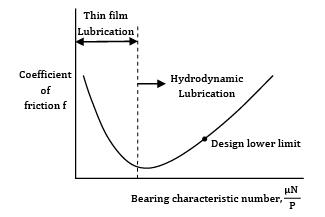QUESTION: 11

A 6308 bearing rotating at 800 rpm is subjected to a dynamic load of 20500 N. The static and dynamic load ratings are 22400 N and 41000 N respectively. The life of bearing in hours is Options~

(A) 124.4

(B) 166.7

(C) 182.3

(D) 144.3

Solution: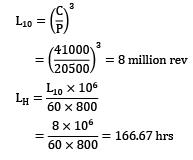QUESTION: 12

A journal bearing of diameter 25 cm and length 40 cm carries a load of 150 kN. The average bearing pressure is

Solution:

The average bearing pressure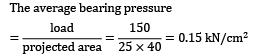QUESTION: 13

A ball bearing operating at a load P has 16000 hours of life. The life of the bearing, in hours, when the load is doubled to 2P is

Solution:

Life of bearing,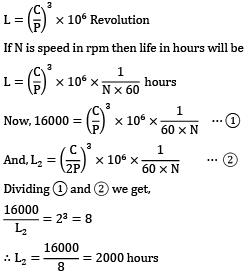If N is speed in rpm then life in hours will beQUESTION: 14

In thick film hydrodynamic journal bearings, the coefficient of friction

Solution: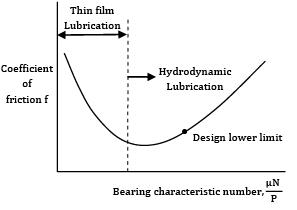QUESTION: 15

Assertion (A): In steady rotating condition the journal inside a hydrodynamic journal bearing remains floating on the oil film.

Reason (R): The hydrodynamic pressure developed in steady rotating conditions in journal bearings balances the load on the journal

Solution:

The film pressure created by the moving surface itself pulling the lubricant into a wedge shaped zone at a velocity sufficiently high to create the necessary pressure required to separate the surface against the load on the bearings. Hydrodynamic lubrication is also called as full film lubrication or fluid lubrication. So, Assertion and Reason both are correct and A is the correct explanation of R.

QUESTION: 16

Radial pressure in a hydrodynamic bearing is

Solution: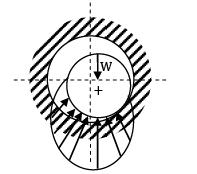Variation of pressure in the converging film.

QUESTION: 17

The following data is given for a hydrodynamic bearing:

Journal speed 1500rpm

Clearance ratio = 850

viscosity = 35centipoise

Journal diameter = 100 mm

Assuming a square journal bearing, the Sommerfeld’s number is

Solution:

Sommerfeld’s number is given by,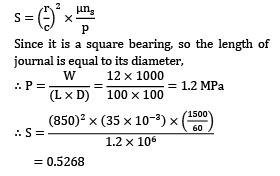Since it is a square bearing, so the length of journal is equal to its diameter,= 0.5268

QUESTION: 18

In a hydrodynamic journal bearing, there

Solution:

In the running condition, there is always a thick film of lubricant between journal and bearing.

*Answer can only contain numeric values
QUESTION: 19

A shaft of 120 mm diameter rotates at 100 rpm in a 200 mm long bearing. The angular space between the shaft and bearing is filled with oil of viscosity 0.7 poise. If the thickness of oil film is 0.075 mm, the power absorbed in the bearing is __________ W.

Solution:

Given,Diameter of shaft, D = 120 mm

= 0.12 m

Length of bearing, L = 0.2 m, t = 0.075 mm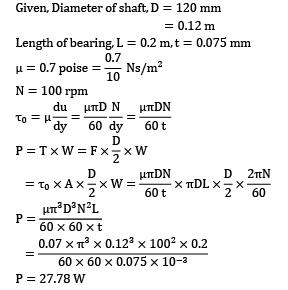N = 100 rpmP = 27.78 W

QUESTION: 20

A taper roller bearing has a dynamic load capacity of 26 kN. The desired life for 90% of the bearings is 8000h and the speed is 300 rpm. The equivalent radial load that the bearing can carry in N is

Solution:

Given,C = 26 kN, L10h = 8000h, N = 300 rpm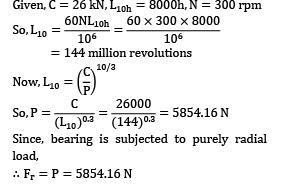144 million revolutions*Answer can only contain numeric values
QUESTION: 21

A journal bearing has journal diameter 50 mm and a bush length of 50 mm. The shaft is rotating at 250 rpm and viscosity of lubricant is 0.4 poise. The power loss (in W) will be __________. (Take clearance = 0.02 mm)

Solution:

Tangential velocity of shaft,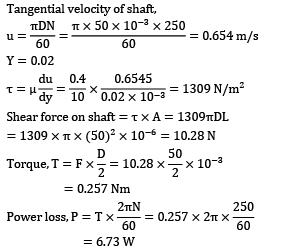Y = 0.02Shear force on shaft = T x A = 1309πDL

= 1309 x π x (50)2 x 10-6 = 10.28 N= 0.257 Nm= 6.73 W

*Answer can only contain numeric values
QUESTION: 22

If load of a ball bearing is decreased from 12kN to 7.25 kN, life of the ball bearing will increased by __________ times.

Solution:

For ball bearing life is given by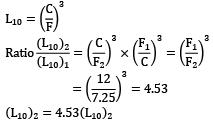(L10)2 = 4.53(L10)2

So, life of the ball bearing is increased by

*Answer can only contain numeric values
QUESTION: 23

A roller bearing dynamic load capacity of 30kN. Equivalent radial load that bearing can carry is 6 kN. The speed is 400 rpm. The desired life for 90% of bearings is __________ hours.

Solution: ]

C = 30 kN

P = 6 kN

P(L10)0.3 = C

6 x (L10)0.3 = 30

L10 = 213.75 million rev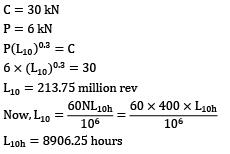L10h = 8906.25 hours

*Answer can only contain numeric values
QUESTION: 24

A full journal square bearing with journal of 100 mm diameter is subjected to a load of 5 kN at 1000 rpm. The lubricant has a viscosity of 12 centipoise, radial clearance is 0.04 mm and eccentricity ratio of the bearing is 0.27. The value of minimum oil thickness is __________ mm. (upto three digits)

Solution:

Attitude or eccentricity ratio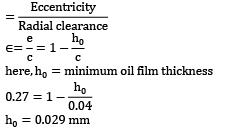here,h0 = minimum oil film thicknessh0 = 0.029 mn

*Answer can only contain numeric values
QUESTION: 25

The following data refers to a 360hydrodynamic bearing:

Journal speed = 1500 r m

Journal diameter = 70 mm

Bearing Length = 100 mm

Lubricant viscosity = 30 centipoise

The Sommerfeld’s number for the bearing is __________.

Solution:

Given,W = 3 kN,N = 1500 rpm,d= 70 mm,

l = 100 mm,c = 0.05,z = 30 centipose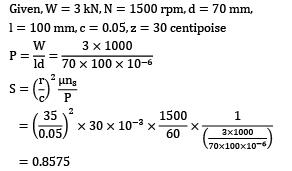= 0.8575

*Answer can only contain numeric values
QUESTION: 26

A deep groove ball bearing has a dynamic load capacity of 40000 N. Work cycle consists of 5000 N at 1440 rpm for 20% time, 3000 N at 2880 rpm for 50% time and 6000 N at 720 rpm for 30% time. The life of bearing is __________. (in million rev)

Solution:

Consider a work cycle of 1 min duration,

N1 = 1440 x 0.2 = 288 revolutions

N2 = 2880 x 0.5 = 1440 revolutions

N3 = 720 x 0.3 = 216 revolutions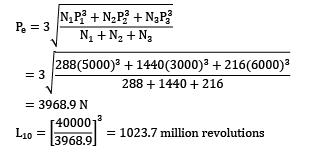= 3968.9 NQUESTION: 27

A ball bearing is operated on a work cycle consisting of three parts: a radial load of at 1440 rpm for one quarter cycle, a radial load of 5000 N at 720 rpm for one half of cycle and a radial load of 2500 N at 1440 rpm for the remaining cycle. The expected life of the bearings is 10, 000 hrs. The dynamic load carrying capacity of the bearings is

Solution: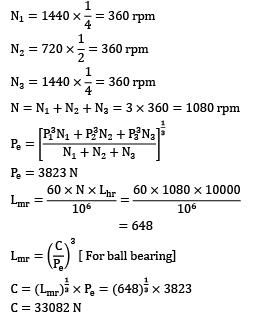N = N1 + N2 + N3 = 3 x 360 = 1080 rpmPe = 3823 NC = 33082 N

*Answer can only contain numeric values
QUESTION: 28

A bearing of 50 mm in diameter has a shaft speed of 320 rpm and a lubricating oil of viscosity 0.06 kg/m.s. The diametral clearance is 0.15 mm and the bearing pressure is 1.5 N/mm2 . If it is designed for a speed of 320 rpm and pressure of 1.5 N/2mm . Clearance of 0.12 mm, the change made for the viscosity is (in kg/m.s) is __________.

Solution: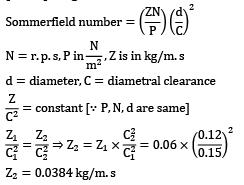d = diameter, C = diametral clearanceZ2 = 0.0384 kg/m.s

QUESTION: 29

A 25 mm radius and 300 mm long shaft is supported at the ends by two journal bearings. A load of 2 kN acts on the shaft at a distance of 200 mm from the left bearing. The length to diameter ratio for the bearings is 1.5. The maximum bearings pressure induced is

Solution: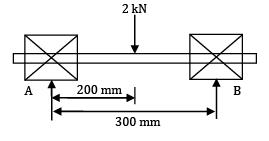From equilibrium conditions,

RA + RB = 2 kN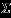MA = 0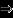RB x 300 = 2 x 200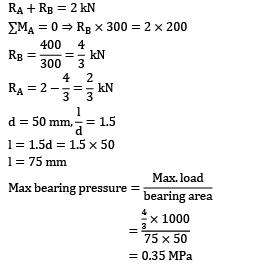l = 1.5d = 1.5 x 50

l = 75 mm= 0.35 MPa

QUESTION: 30

A system involves four identical ball bearings, each subjected to a radial load of 2500 N. The reliability of the system, i.e., one out of four bearings failing during the lifetime of five million revolutions, is 82%. The dynamic load carrying capacity of the bearing, so as to select it from the manufacturer’s catalogue based on 90% reliability will be

Solution:

P = 2500 N

Reliability of the system = 0.82

Hence reliability of each bearing = [0.82]¼

= 0.95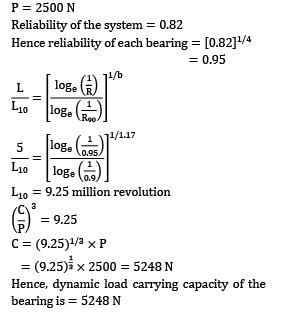L10 = 9.25 million revolutionC = (9.25)⅓. X P

= (9.25) x 2500 = 5248

Hence, dynamic load carrying capacity of the bearing is = 5248 N